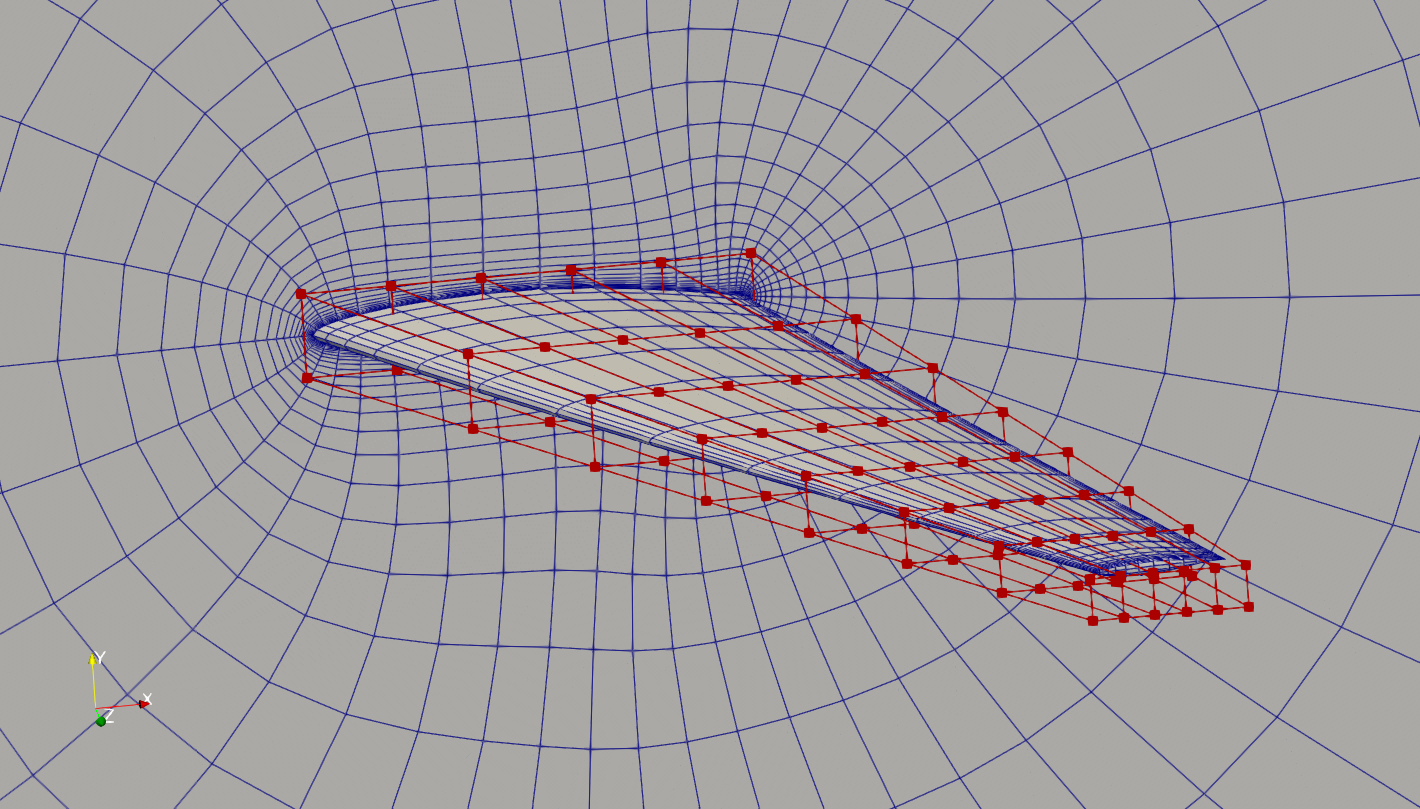The following is an aerostructural shape optimization case for the MACH tutorial wing in subsonic conditions. The flow is solved using the DARhoSimpleFoam CFD solver and the structure is solved using an open-source FEM solver TACS. The load and displacement transfer is computed using FUNtoFEM. The aerostructural coupling is implemented in the OpenMDAO/Mphys framework.

```Case: Wing aerostructural optimization
Geometry: MACH tutorial wing
Objective function: Drag coefficient (CD)
Lift coefficient (CL): 0.5
Design variables: 96 FFD points moving in the y direction, seven twists, and one angle of attack.
Constraints: volume, thickness, LE/TE, lift, and stress constraints (total number: 118)
Mach number: ~0.3 (100 m/s)
Reynolds number: ~30 million
Mesh cells: ~38,000
Solver: DARhoSimpleFoam
```Fig. 1. Mesh and FFD points for the MACH tutorial wing.

To run this case, first download tutorials and untar it. Then go to tutorials-main/MACH_Tutorial_Wing and run the “preProcessing.sh” script to generate the mesh:

```./preProcessing.sh
```

The above script will generate a structured hex mesh using pyHyp. Then, use the following command to run the optimization with 8 CPU cores:

```mpirun -np 8 python runScript_AeroStruct.py 2>&1 | tee logOpt.txt
```